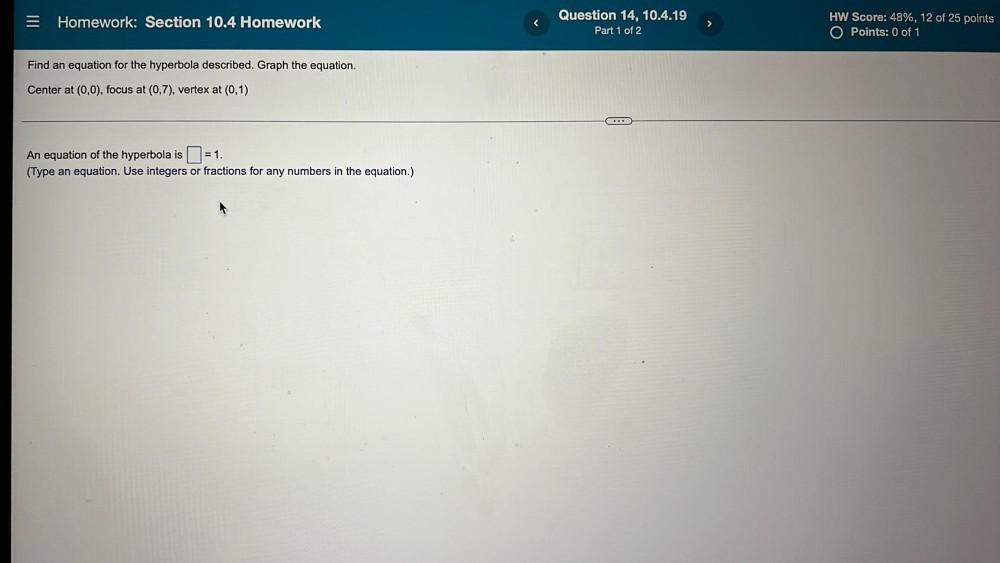Question:

# = Homework: Section 10.4 Homework Question 14, 10.4.19 Part 1 of 2 HW Score: 48%, 12 of 25 points O Points: 0 of 1 Find an equat= Homework: Section 10.4 Homework Question 14, 10.4.19 Part 1 of 2 HW Score: 48%, 12 of 25 points O Points: 0 of 1 Find an equation for the hyperbola described. Graph the equation. Center at (0,0), focus at (0,7), vertex at (0,1) ... An equation of the hyperbola is 1=1. (Type an equation. Use integers or fractions for any numbers in the equation.)